## Saturday, February 23, 2013

### No. 44 - Dynamic Programming on Stolen Values

Problem: There are n houses built in a line, each of which contains some value in it. A thief is going to steal the maximal value in these houses, but he cannot steal in two adjacent houses because the owner of a stolen house will tell his two neighbors on the left and right side. What is the maximal stolen value?

For example, if there are four houses with values {6, 1, 2, 7}, the maximal stolen value is 13 when the first and fourth houses are stolen.

Analysis: A function f(i) is defined to denote the maximal stolen value from the first house to the ith house, and the value contained in the ith house is denoted as vi. When the thief reaches the ith house, he has two choices: to steal or not. Therefore, f(i) can be defined with the following equation:
It would be much more efficient to calculate in bottom-up order than to calculate recursively. It looks like a 1D array with size n is needed, but actually it is only necessary to cache two values for f(i-1) and f(i-2) to calculate f(i).

This algorithm can be implemented with the following C++ code:

int maxStolenValue(const vector<int>& values)
{
int length = values.size();
if(length == 0)
return 0;

int value1 = values;
if(length == 1)
return value1;

int value2 = max<int>(values, values);
if(length == 2)
return value2;

int value;
for(int i = 2; i < length; ++i)
{
value = max<int>(value2, value1 + values[i]);
value1 = value2;
value2 = value;
}

return value;
}

More coding interview questions are discussed in my book <Coding Interviews: Questions, Analysis & Solutions>. You may find the details of this book on Amazon.com, or Apress.

The author Harry He owns all the rights of this post. If you are going to use part of or the whole of this ariticle in your blog or webpages, please add a reference to http://codercareer.blogspot.com/. If you are going to use it in your books, please contact him via zhedahht@gmail.com . Thanks.

## Friday, February 22, 2013

### No. 43 - Minimal Number of Palindromes on a String

Problem: A string can be partitioned into some substrings, such that each substring is a palindrome. For example, there are a few strategies to split the string “abbab” into palindrome substrings, such as: “abba”|”b”, “a”|”b”|”bab” and “a”|”bb”|”a”|”b”.

Given a string str, please get the minimal numbers of splits to partition it into palindromes. The minimal number of splits to partition the string “abbab” into a set of palindromes is 1.

Analysis: This is a typical problem which can be solved by dynamic programming. We have two strategies to analyze and solve this problem

Solution 1: Split at any space between two characters

Given a substring of str, starting from the index i and ending at the index j (denoted as str[i:j]), we define a function f(i, j) to denote the minimal number of splits to partition the substring str[i:j] into a set of palindromes. If the substring is a palindrome itself, we don’t have to split so f(i, j) is 0. If the substring is not a palindrome, the substring is split between two characters k and k+1. f(i, j)= f(i, k)+ f(k+1, j)+1 under such conditions. Therefore, f(i, j) can be defined with the following equation: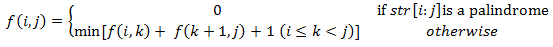The value of f(0, n-1) is the value of the minimal number of splits to partition str into palindromes, if n is the length of str.

If the equation is calculated recursively, its complexity grows exponentially with the length n. A better choice is to calculate in bottom-up order with a 2D matrix with size n×n. The following C++ code implements this solution:

int minSplit_1(const string& str)
{
int length = str.size();
int* split = new int[length * length];

for(int i = 0; i < length; ++i)
split[i * length + i] = 0;

for(int i = 1; i < length; ++i)
{
for(int j = length - i; j > 0; --j)
{
int row = length - i - j;
int col = row + i;
if(isPalindrome(str, row, col))
{
split[row * length + col] = 0;
}
else
{
int min = 0x7FFFFFFF;
for(int k = row; k < col; ++k)
{
int temp1 = split[row * length + k];
int temp2 = split[(k + 1) * length + col];
if(min > temp1 + temp2 + 1)
min = temp1 + temp2 + 1;
}
split[row * length + col] = min;
}
}
}

int minSplit = split[length - 1];
delete[] split;
return minSplit;
}

Solution 2: Split only before a palindrome

We split the string str with another strategy. Given a substring ending at the index i, str[0, i], we do not have to split if the substring is a palindrome itself. Otherwise it is split between two characters at index j and j+1 only if the substring str[j+1,i] is a palindrome. Therefore, an equation f(i) can be defined as the following:The value of f(n-1) is the value of the minimal number of splits to partition str into palindromes, if n is the length of str.

We could utilize a 1D array to solve this equation in bottom-up order, as listed in the following code:

int minSplit_2(const string& str)
{
int length = str.size();
int* split = new int[length];
for(int i = 0; i < length; ++i)
split[i] = i;

for(int i = 1; i < length; ++i)
{
if(isPalindrome(str, 0, i))
{
split[i] = 0;
continue;
}

for(int j = 0; j < i; ++j)
{
if(isPalindrome(str, j + 1, i) && split[i] > split[j] + 1)
split[i] = split[j] + 1;
}
}

int minSplit = split[length - 1];
delete[] split;
return minSplit;
}

Optimization to verify palindromes:

Usually it costs O(n) time to check whether a string with length n is a palindrome, and the typical implementation looks like the following code:

bool isPalindrome(const string& str, int begin, int end)
{
for(int i = begin; i < end - (i - begin); ++i)
{
if(str[i] != str[end - (i - begin)])
return false;
}

return true;
}

Both solutions above cost O(n3) time. The first solution contains three nesting for-loops. The function isPalindrome is inside two nesting for-loops.

If we could reduce the cost of isPalindrome to O(1), the time complexity of the second solution would be O(n2).

Notice that the substring str[i,j] is a palindrome only if the characters at index i and j, and str[i+1,j-1] is also a palindrome. We could build a 2D table accordingly to store whether every substring of str is a palindrome or not during the preprocessing. With such a table, the function isPalindrome can verify the substring str[i,j] in O(1) time.

More coding interview questions are discussed in my book <Coding Interviews: Questions, Analysis & Solutions>. You may find the details of this book on Amazon.com, or Apress.

The author Harry He owns all the rights of this post. If you are going to use part of or the whole of this ariticle in your blog or webpages, please add a reference to http://codercareer.blogspot.com/. If you are going to use it in your books, please contact him via zhedahht@gmail.com . Thanks.

## Thursday, February 21, 2013

### No. 42 - Three Increasing Elements in an Array

Problem: Given an array of integers A, please find three indexes i, j, k, such that i<j<k and A[i]<A[j]<A[k].

Analysis: Firstly let’s analyze this problem with the brute-force solution: All elements in the array are scanned one by one. When an element is scanned, we take is as A[j], and check whether there is a smaller element on its left side (A[i]), and a greater element on its right side (A[k]).
Since it takes O(n) time to scan numbers on two sides of each A[j], the overall time of this solution is O(n2) on an array with n elements.

Solution 1: O(n) time efficiency with O(n) space consumption
As our analysis above, for each element A[j], we need to know whether there is a smaller element on its left side. If there is not a smaller element on the left side, A[j] itself is the smallest of all elements from the leftmost element to A[j]. If there is a smaller element on the left side, the smallest of all elements from the leftmost element to A[j] is on the left side of A[j].

Therefore, we could construct an auxiliary array B. The element B[j] stores the index of the smallest element of elements from the leftmost element to A[j]. The array B can be constructed based on elements in A from left to right.

Similarly, we could also construct another auxiliary array C. The element C[j] stores the index of the greatest element of elements from the rightmost element to A[j]. The array C can be constructed based on elements in A from right to left.

When an element A[j] is scanned, the index j is compared with B[j] and C[j]. if B[j]<j (there is a smaller element on the left side) and j<C[j] (there is a greater element on the right side), three increasing elements have been found.

This solution can be implemented with the following C/C++ code:

void increasingIndex_1(int* nums, int length, int* i, int* j, int* k)
{
if(nums == NULL || length <= 0 || i == NULL || j == NULL || k == NULL)
return;

int* minIndex = new int[length];
int index = 0;
minIndex = 0;
int t;
for(t = 1; t < length; ++t)
{
if(nums[t] < nums[index])
index = t;
minIndex[t] = index;
}

int* maxIndex = new int[length];
index = length - 1;
for(t = length - 1; t >= 0; --t)
{
if(nums[t] > nums[index])
index = t;
maxIndex[t] = index;
}

for(t = 1; t < length - 1; ++t)
{
if(minIndex[t] < t && maxIndex[t] > t)
break;
}
if(t < length - 1)
{
*i = minIndex[t];
*j = t;
*k = maxIndex[t];
}
else
{
*i = *j = *k = -1;
}

delete[] minIndex;
delete[] maxIndex;
}

Solution 2: O(n) time efficiency with O(1) space consumption
In order to find an even more efficient solution, let’s scan all elements in an array and try to find three increasing elements. The array {3, 2, 5, 1, 4, 7, 9, 6, 8} is taken as an example to simulate the process.

At first, all elements A[i], A[j], and A[k] have not been found, so three indexes i, j, and k are initialized as -1.

The number 3 is the first number to be scanned, and A[i] is updated as 3.

The next number to be scanned is the number 2. Notice that 2 is less than 3, and it is a better candidate of A[i]. The less A[i] is, the larger range A[j] and A[k] will have. Therefore, A[i] is updated as 2.

Let’s continue to scan the next number 5. Since 5 is greater the current A[i], it is a candidate of A[j]. A[j] is updated as 5.

The number 1 is scanned in the next round. Because 1 is less than the current A[j], it cannot be A[k]. Additionally, the number 1 is a candidate to be A[i] or A[j] in the future since it is less than the current A[i] and A[j]. However, neither A[i] nor A[j] can be updated as 1 at this time. If A[i] is updated as 1, A[i]<A[j] but i>j. If A[j] is updated as 1, A[i]>A[j]. Therefore, we store the number 1 into another variable named t.

We move on to scan the next number 4. Notice that the number 4 is less than A[j] again. At this time we have two numbers 1 (the stored variable t) and 4, so we have a new pair of numbers to be A[i] and A[j]. A[i] and A[j] are updated as 1 and 4 respectively.

The next number, 7, is greater than the current A[j], so A[k] is updated as 7. The process terminates.
The following table summarizes the step-by-step analysis above:

 Step Scanned Number A[i] A[j] A[k] t Operation 1 3 3 -1 -1 -1 Update A[i] 2 2 2 -1 -1 -1 Update A[i] 3 5 2 5 -1 -1 Update A[j] 4 1 2 5 -1 1 Update t 5 4 1 4 -1 -1 Update A[i], A[j] and t 6 7 1 4 7 -1 Update A[k]. Terminate.

The strategy of this solution is to store as less value to A[i] and A[j] as possible, in order to enlarge the value range for steps in the future.

With our step-by-step analysis, we could implement this solution with the following C++ code:

void increasingIndex_2(int* nums, int length, int* i, int* j, int* k)
{
if(nums == NULL || length <= 0 || i == NULL || j == NULL || k == NULL)
return;

*i = *j = *k = -1;
int backup = -1;

int index;
for(index = 0; index < length; ++index)
{
if(*i == -1)
{
*i = index;
}
else if(*j == -1)
{
if(nums[index] > nums[*i])
*j = index;
else
*i = index;
}
else if(nums[index] > nums[*j])
{
*k = index;
break;
}
else if(nums[index] < nums[*j])
{
if(backup != -1)
{
if(nums[index] > nums[backup])
{
*i = backup;
*j = index;
backup = -1;
}
else if (nums[index] < nums[backup])
{
backup = index;
}
}
else if(nums[index] < nums[*j])
backup = index;
}
}
if(index == length)
*i = *j = *k = -1;
}

More coding interview questions are discussed in my book <Coding Interviews: Questions, Analysis & Solutions>. You may find the details of this book on Amazon.com, or Apress.

The author Harry He owns all the rights of this post. If you are going to use part of or the whole of this ariticle in your blog or webpages, please add a reference to

## Wednesday, February 20, 2013

### No. 41 - Group of 1s in a Matrix

Problem: Given a matrix with 1s and 0s, please find the number of groups of 1s. A group is defined by horizontally or vertically adjacent 1s. For example, there are four groups of 1s in Figure 1 which are drawn with different colors.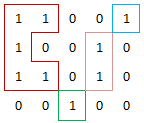Figure 1: A matrix with four groups of 1s. Different groups are drawn with different colors.

Analysis: All numbers in the matrix are scanned one by one. When a 1 is met, a group of 1s has been found, and then all 1s in the group are flipped to 0s.
For example, the first entry in the matrix of Figure 1 contains 1, so the first group of 1s is found when we access to the first entry. After all 1s in the group have been flipped to 0s, the matrix becomes the one in Figure 2.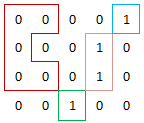Figure 2: The new matrix after all 1s in the first group (in the red boundary) have been flipped

Therefore, the overall function to count the groups of 1s in a matrix can be implemented in the following C++ code:
int groupOf1(int* nums, int rows, int cols)
{
if(nums == NULL || rows <= 0 || cols <= 0)
return 0;

int group = 0;
for(int i = 0; i < rows * cols; ++i)
{
if(nums[i] == 1)
{
group++;
}
}

return group;
}

Let’s move on to flip 1s inside a group. Actually, it is an application of the seed filling algorithm. When we are going to flip a group starting from an entry, we take the entry as a seed and push it into a stack. At each step, we get an entry from the top of the stack, flip it, and then push its neighboring entries into the stack. The process continues until the stack is empty. The following code implements this algorithm:
void flipAdjacent1(int* nums, int rows, int cols, int index)
{
stack<int> group;
group.push(index);
while(!group.empty())
{
int topIndex = group.top();
group.pop();
nums[topIndex] = 0;

int row = topIndex / cols;
int col = topIndex % cols;

// up
if(row > 0 && nums[(row - 1) * cols + col] == 1)
group.push((row - 1) * cols + col);
// right
if(col < cols - 1 && nums[row * cols + col + 1] == 1)
group.push(row * cols + col + 1);
// down
if(row < rows - 1 && nums[(row + 1) * cols + col] == 1)
group.push((row + 1) * cols + col);
// left
if(col > 0 && nums[row * cols + col - 1] == 1)
group.push(row * cols + col - 1);
}
}

More coding interview questions are discussed in my book <Coding Interviews: Questions, Analysis & Solutions>. You may find the details of this book on Amazon.com, or Apress.

The author Harry He owns all the rights of this post. If you are going to use part of or the whole of this ariticle in your blog or webpages, please add a reference to

## Monday, February 18, 2013

### No. 40 - Add on Lists

Problem: Nodes in a list represent a number. For example, the nodes in Figure 1 (a) and (b) represent numbers 123 and 4567 respectively. Please implement a function/method to add numbers in two lists, and store the sum into a new list.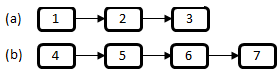Figure 1: Two lists representing numbers. (a) A list for 123; (b) A list for 4567.
Analysis: Usually numbers are added beginning from the least significant digits (The digit 3 in the number 123, and the digit 7 in the number 4567). As shown in Figure 1, the least significant digits are at the tail of lists, and they can be accessed after the whole lists are scanned. Therefore, lists should be reversed at first, in order get the least significant digits before other digits. The two reversed lists of lists in Figure 1 are shown in Figure 2.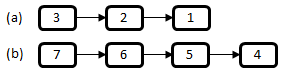Figure 2: Two reversed lists of the lists in Figure 1.

After two lists are reversed, we can add nodes along the links between nodes, and then reversed the result list after all nodes are added. Therefore, the overall structure to add numbers in two lists can be implemented with the following code in C/C++:

{
return NULL;

return Reverse(pResult);
}

Now let’s implement the function AddReversed, to add nodes in two reversed lists. Digits are gotten in nodes along links between nodes. When we get two digits in two lists, we add them and create a new node to store the sum, and append the new node into the list for result. There are two issues worthy of attention: (1) The length of two lists might be different; (2) The sum of two digits may be greater than 10, so we have to take care of the carry when adding two digits. The function AddReversed can be implemented with the following C/C++ code:

{
int carry = 0;
ListNode* pPrev = NULL;
{

}
if(carry > 0)
{
ListNode* pNode = CreateListNode(carry);
}

}

The function AddNode adds digits in two nodes. The third parameter of this function takes the carry for addition calculation, as listed below:

ListNode* AddNode(ListNode* pNode1, ListNode* pNode2, int* carry)
{
int num1 = 0;
if(pNode1 != NULL)
num1 = pNode1->m_nValue;
int num2 = 0;
if(pNode2 != NULL)
num2 = pNode2->m_nValue;

int sum = num1 + num2 + *carry;
*carry = (sum >= 10) ? 1 : 0;

int value = (sum >= 10) ? (sum - 10) : sum;
return CreateListNode(value);
}

The function AppendNode is used append a node into the tail of lists. In order to avoid scanning the whole list to get the previous tail every time, the previous tails is stored in the parameter/variable pPrev, as listed in the following code:

void AppendNode(ListNode** pHead, ListNode** pPrev, ListNode* pNode)
{
if(*pPrev == NULL)
*pPrev = pNode;
else
{
(*pPrev)->m_pNext = pNode;
*pPrev = pNode;
}
}

The function CreateListNode is to create a list node according to a value, which is omitted here because it’s quite straightforward.

The steps to reverse a list are discussed in my previous blog.

More coding interview questions are discussed in my book <Coding Interviews: Questions, Analysis & Solutions>. You may find the details of this book on Amazon.com, or Apress.

The author Harry He owns all the rights of this post. If you are going to use part of or the whole of this ariticle in your blog or webpages, please add a reference to